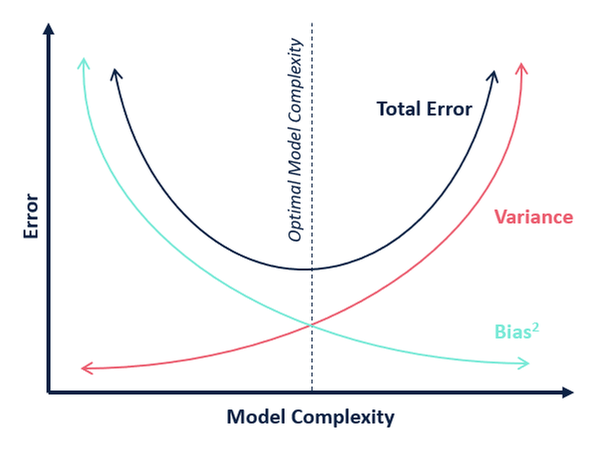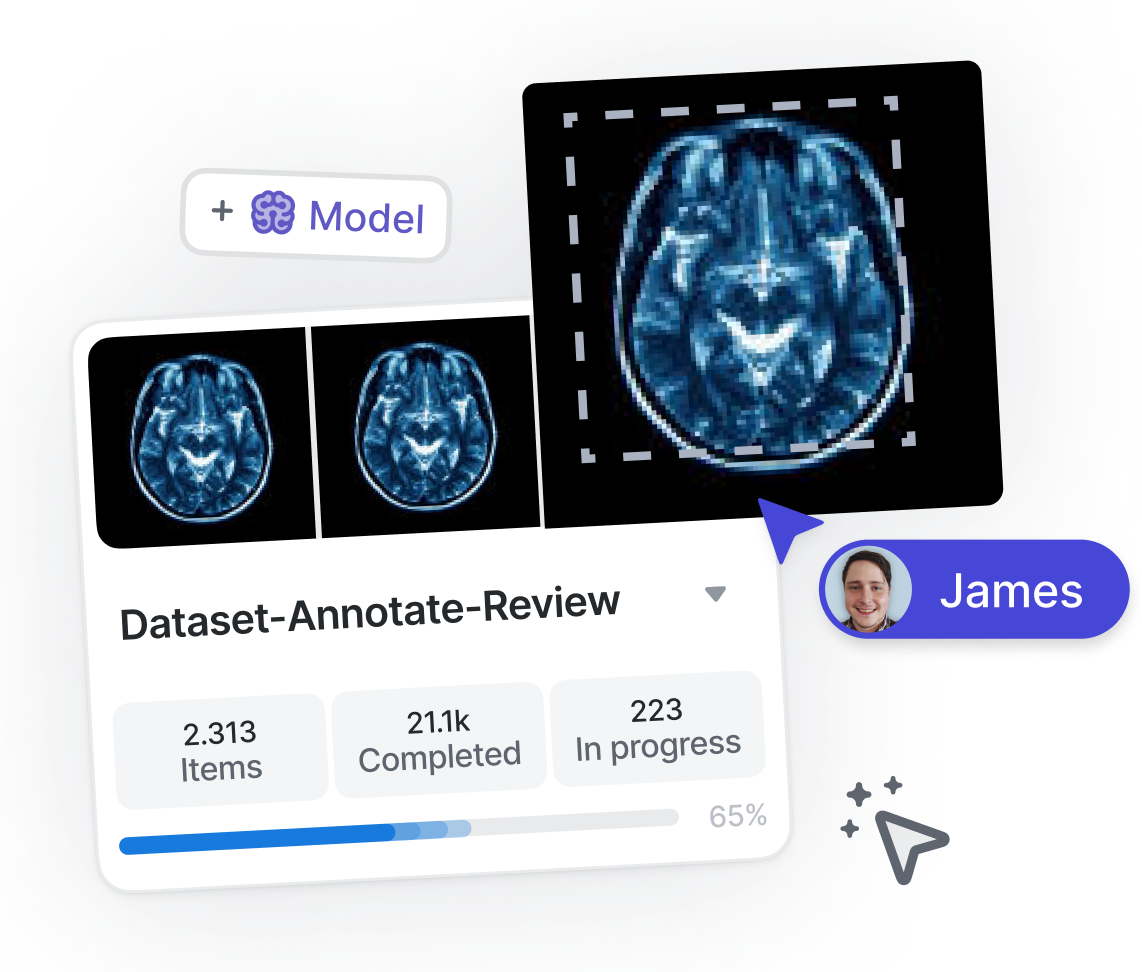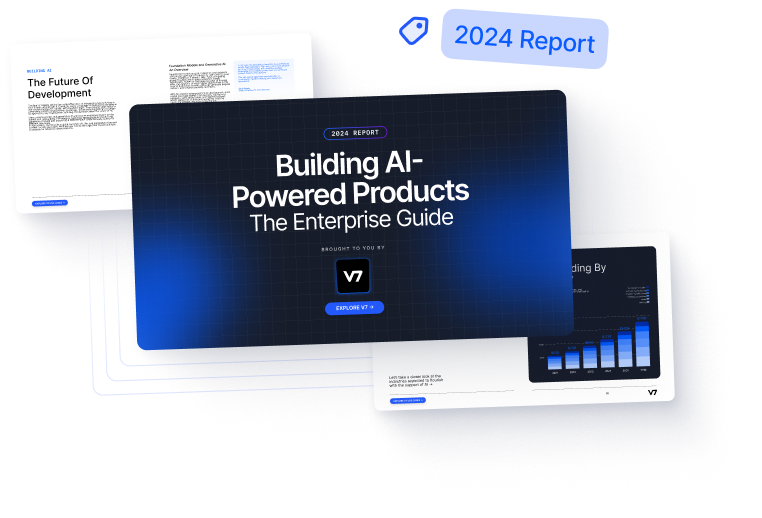# Overfitting vs. Underfitting: What's the Difference?

What's the difference between overfitting and underfitting? How can you prevent those modeling errors from harming the performance of your model? Read to find out and use V7 to build AI models that don't suck.9
January 31, 2022Here’s something that no data scientist wants to hear—

One will never compose a perfect dataset with balanced class distributions, no noise and outliers, and uniform data distribution in the real world.

That means that our model has slim chances of becoming infallible, but we still want it to describe the underlying patterns – and do it correctly.

Unfortunately—

It’s not that simple.

The nature of data is that it comes with some noise and outliers even if, for the most part, we want the model to capture only the relevant signal in the data and ignore the rest.

Both underfitting and overfitting of the model are common pitfalls that you need to avoid.

In this article, we’ll have a deeper look at those two modeling errors and suggest some strategies to ensure that they don’t hinder your model’s performance.

Here’s what we’ll cover:

1. The importance of Generalization in Machine Learning
2. What is Overfitting in Machine Learning?
3. What is Underfitting in Machine Learning?
4. A Good Fit in Machine Learning
5. How to avoid Overfitting?
6. How to reduce Underfitting?

Train ML models and solve any computer vision task faster with V7.

Don't start empty-handed. Explore our repository of 500+ open datasets and test-drive V7's tools.

Ready to streamline AI product deployment right away? Check out:

## Generalization in Machine Learning

Machine learning can be described as the process of “generalizing from examples.”

Generalization in machine learning is used to measure the model’s performance to classify unseen data samples. A model is said to be generalizing well if it can forecast data samples from diversified sets.

For the model to generalize, the learning algorithm needs to be exposed to different subsets of data.

Two major things limit generalization:

1. The crux of the model is the data samples we feed into the model for the system to learn.
2. Behind the scenes learning algorithm used by the model that determines how well the knowledge is generalized.

In the case of supervised learning, the model aims to predict the target function(Y) for an input variable(X). If the model generalizes the knowledge, the prediction variable(Y') would be naturally close to the ground truth.

Sounds a little complicated?

Statistical fit: The goodness of the fit is the statistical technique to measure how well the data points fit the model’s curve. The model is a good fit if it converges well between the expected and observed data points.

Training error: The fitness of the predictive model is calculated by comparing the actual and expected values. When this is done on the training data itself, it gives us the training error.

Test error: Predictive models find the mapping between the input variable and the target value. The corresponding error is called test error when we test the model on an unknown dataset.

Bias: It measures the difference between the model’s prediction and the target value. If the model is oversimplified, the predicted value would be far from the ground truth resulting in more bias.

Variance: Variance measures the inconsistency of different predictions over a varied dataset. Suppose the model's performance is tested on different datasets—the closer the prediction, the lesser the variance. Higher variance indicates overfitting, in which the model loses the ability to generalize.

## What is Overfitting in Machine Learning?

Overfitting is a common pitfall in deep learning algorithms, in which a model tries to fit the training data entirely and ends up memorizing the data patterns and the noise/random fluctuations. These models fail to generalize and perform well in the case of unseen data scenarios, defeating the model's purpose.

Signs of overfitting

Detecting overfitting is only possible once we move to the testing phase. Overfitted models can’t generalize datasets.

The most “primitive” way to start the process of detecting overfitting in machine learning models is to divide the dataset so that we can examine the model's performance on each set of data individually.

But there are also other options—

K-fold cross-validation is one of the most common techniques used to detect overfitting. Here, we split the data points into k equally sized subsets in K-folds cross-validation, called "folds." One split subset acts as the testing set while the remaining groups are used to train the model.

##### 💡 Pro tip: Looking for a quality data? Check out 65+ Best Free Datasets for Machine Learning and 20+ Open Source Computer Vision Datasets.

The model is trained on a limited sample to assess how it would perform in general when used to make predictions on the unseen data. One fold acts as a validation set in each turn. After all the iterations, we average the scores to assess the performance of the overall model.

Overfitting happens when:

1. The training data is not cleaned and contains some “garbage”     values. The model captures the noise in the training data and fails to generalize the model's learning.
2. The model has a high variance.
3. The training data size is insufficient, and the model trains on the limited training data for several epochs.
4. The architecture of the model has several neural layers bundled together. Deep neural networks are complex and require a significant amount of time to train, and often lead to overfitting the training set.
5. Incorrect tuning of hyperparameters in the training phase leads to over-observing the training set, resulting in memorizing features.

## What is Underfitting in Machine Learning

Underfitting is another common pitfall in machine learning, where the model cannot create a mapping between the input and the target variable. Under-observing the features leads to a higher error in the training and unseen data samples.

It is different from overfitting, where the model performs well in the training set but fails to generalize the learning to the testing set.

Underfitting becomes obvious when the model is too simple and cannot create a relationship between the input and the output. It is detected when the training error is very high and the model is unable to learn from the training data. High bias and low variance are the most common indicators of underfitting.

Underfitting happens when:

1. Unclean training data containing noise or outliers can be a reason for the model not being able to derive patterns from the dataset.
2. The model has a high bias due to the inability to capture the relationship between the input examples and the target values. This usually happens in the case of varied datasets.
3. The model is assumed to be too simple—for example, we train a linear model in complex scenarios.
4. Incorrect hyperparameters tuning often leads to underfitting due to under-observing of the features.

## A Good Fit in Machine Learning

A good fit is when the machine learning model achieves a balance between bias and variance and finds an optimal spot between the underfitting and overfitting stages. The goodness of fit, in statistical terms, means how close the predicted values match the actual values.

There are a couple of techniques to achieve a good fit:

### 1. Introduction of the validation set

Earlier, a test set was used to validate the model's performance on unseen data. A validation dataset is a sample of data held back from training your model to tune the model’s hyperparameters. It estimates the performance of the final—tuned—model when selecting between final models.

### 2. Resampling methods

Resampling is a technique of repeated sampling in which we take out different samples from the complete dataset with repetition. The model is trained on these subgroups to find the consistency of the model across different samples. Resampling techniques build the confidence that the model would perform optimally no matter what sample is used for training the model.

Manage your datasets and train models 10x faster

Keep all your training data in one place. Curate, browse and visualize millions of items across your organization.## How to avoid Overfitting?

Here we will discuss possible options to prevent overfitting, which helps improve the model performance.

### 1. Train with more data

With the increase in the training data, the crucial features to be extracted become prominent. The model can recognize the relationship between the input attributes and the output variable.

The only assumption in this method is that the data to be fed into the model should be clean; otherwise, it would worsen the problem of overfitting.

### 2. Data augmentation

An alternative method to training with more data is data augmentation, which is less expensive and safer than the previous method. Data augmentation makes a sample data look slightly different every time the model processes it.

### 3. Addition of noise to the input data

Another option (similar to data augmentation) is adding noise to the input and output data.

Adding noise to the input makes the model stable without affecting data quality and privacy, while adding noise to the output makes the data more diverse. Noise addition should be done carefully so that it does not make the data incorrect or irrelevant.

### 4. Feature selection

Every model has several parameters or features depending upon the number of layers, number of neurons, etc.  The model can detect many redundant features leading to unnecessary complexity. We now know that the more complex the model, the higher the chances of the model to overfit.

### 5. Cross-validation

As mentioned above, cross-validation is a robust measure to prevent overfitting. The complete dataset is split into parts.

In standard K-fold cross-validation, we need to partition the data into k folds. Then, we iteratively train the algorithm on-1 folds while using the remaining holdout fold as the test set. This method allows us to tune the hyperparameters of the neural network or machine learning model and test it using completely unseen data.

### 6. Simplify data

Till now, we have come across model complexity to be one of the top reasons for overfitting. The data simplification method is used to reduce overfitting by decreasing the complexity of the model to make it simple enough that it does not overfit.

Some of the procedures include pruning a decision tree, reducing the number of parameters in a neural network, and using dropout on a neutral network.

### 7. Regularization

If overfitting occurs when a model is too complex, reducing the number of features makes sense. Regularization methods like Lasso, L1 can be beneficial if we do not know which features to remove from our model.

Regularization applies a "penalty" to the input parameters with the larger coefficients, which subsequently limits the model's variance.

### 8. Ensembling

It is a machine learning technique that combines several base models to produce one optimal predictive model. InEnsemble Learning, the predictions are aggregated to identify the most popular result.

Well-known ensemble methods include bagging and boosting, which prevents overfitting as an ensemble model is made from the aggregation of multiple models.

### 9. Early stopping

This method aims to pause the model's training before memorizing noise and random fluctuations from the data.

There can be a risk that the model stops training too soon, leading to underfitting. One has to come to an optimum time/iterations the model should train.

Large weights in a neural network signify a more complex network.

Probabilistically dropping out nodes in the network is a simple and effective method to prevent overfitting. In regularization, some number of layer outputs are randomly ignored or “dropped out” to reduce the complexity of the model.

## How to reduce Underfitting?

You already know that underfitting harms the performance of your model. To avoid underfitting, we need to give the model the capability to enhance the mapping between the dependent variables.

Below are a few methods that reduce the problem of underfitting.

### 1. Decrease regularization

Regularization discourages learning a more complex model to reduce the risk of overfitting by applying a penalty to some parameters. L1 regularization, Lasso regularization, and dropout are methods that help reduce the noise and outliers within a model.

More complexity is introduced into the model by decreasing the amount of regularization, allowing for successful model training.

### 2. Increase the duration of training

Early stopping the training can result in the underfitting of the model. There must be an optimal stop where the model would maintain a balance between overfitting and underfitting.

### 3. Feature selection

Introducing more features helps make the model more predictive. For example, we might introduce more hidden layers in deep neural networks, or in machine learning algorithms like the random forest, we may add more dependent variables.

This process will inject more complexity into the model, yielding better training results.

### 4. Remove noise from data

Removing noise from the training data is one of the other methods used to avoid underfitting. The presence of garbage values and outliers often cause underfitting, which can be removed by applying data cleaning and preprocessing techniques on the data samples.

## Key takeaways: Overfitting vs.Underfitting

• Overfitting and underfitting are the two biggest causes of the poor performance of machine learning algorithms and models.
• The scenario in which the model performs well in the training phase but gives a poor accuracy in the test dataset is called overfitting.
• The scenario in which the model performs poorly on the training dataset as it cannot derive features from the training set.
• To achieve a good fit means to stop training the model at an optimal point such that the model is neither under observing the features nor learning the unnecessary details and the noise in the training set.

The Definitive Guide to Instance Segmentation [+V7 Tutorial]

The Beginner’s Guide to Semantic Segmentation

YOLO: Real-Time Object Detection Explained

The Beginner's Guide to Self-Supervised Learning

The Ultimate Guide to Semi-Supervised Learning

9 Reinforcement Learning Real-Life Applications

Mean Average Precision (mAP) Explained: Everything You Need to Know

The Beginner's Guide to Deep Reinforcement Learning

The Complete Guide to CVAT—Pros & ConsPragati is a software developer at Microsoft, and a deep learning enthusiast. She writes about the fundamental mathematics behind deep neural networks.

“Collecting user feedback and using human-in-the-loop methods for quality control are crucial for improving Al models over time and ensuring their reliability and safety. Capturing data on the inputs, outputs, user actions, and corrections can help filter and refine the dataset for fine-tuning and developing secure ML solutions.”Name
Company
GUIDE
Building AI-Powered Products: The Enterprise Guide

Building AI products? This guide breaks down the A to Z of delivering an AI success story.

Oops! Something went wrong while submitting the form.## Related articles

Gain control of your training data
15,000+ ML engineers can’t be wrongGUIDE
FREE
Building AI-Powered Products: The Enterprise Guide
A Comprehensive Guide

Building AI products? This guide breaks down the A to Z of delivering an AI success story.